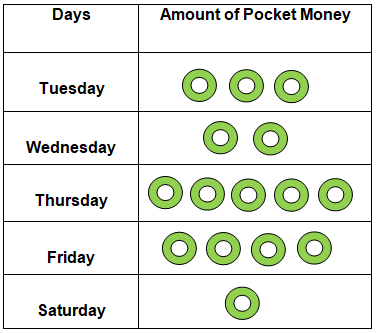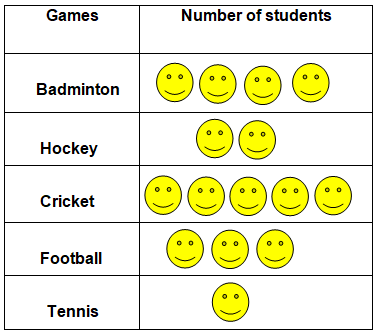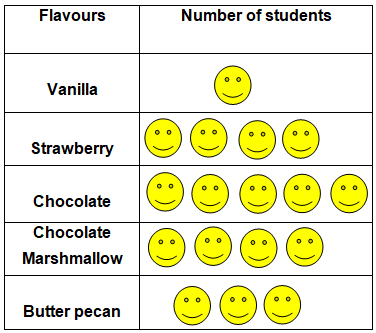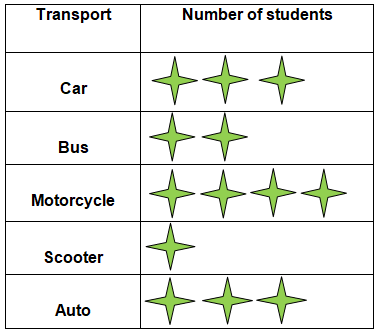# Data handling Worksheets for Grade 3

Download Data handling Worksheets for Grade 3Q.1) The following pictograph shows the number of bananas Aman and Mohit ate during the week-
Each symbol = 2 bananas

i) Find the number of bananas they ate on Tuesday ?

[A] 6

[B] 4

[C] 8

ii) Find the number of bananas they ate on Monday ?

[A] 6

[B] 5

[C] 3

iii) Find the number of bananas they ate on Wednesday ?

[A] 6

[B] 10

[C] 8

iv) Find the total number of bananas they ate on Sunday, Wednesday and Thursday?

[A] 20

[B] 30

[C] 10

v) On which day maximum number of bananas were eaten ?

[A] Monday

[B] Thursday

[C] Wednesday

Q.2) The following pictograph shows how much pocket money Sanjay earned every day from Tuesday to Saturday –

Each symbol = ₹ 5i) How much money did Sanjay earn on Tuesday ?

[A] ₹ 18

[B] ₹ 14

[C] ₹ 15

ii) How much money did Sanjay earn on Friday ?

[A] ₹ 20

[B] ₹ 25

[C] ₹ 18

iii) On which day did he earn the maximum money ?

[A] Saturday

[B] Friday

[C] Thursday

iv) ​What is the total amount earned by him on Thursday, Friday and Saturday ?

[A] ₹60

[B] ₹50

[C] ₹70

v) On which day did he earn the minimum money ?

[A] Friday

[B] Wednesday

[C] Saturday

Q.3) The following pictograph shows how many students of a class like which of the games –

Each symbol = 6 Studentsi) ​How many students like Football ?

[A] 18

[B] 20

[C] 22

ii) How many students like Cricket ?

[A] 60

[B] 30

[C] 20

iii) Which game is liked the most by the students ?

[A] Tennis

[B] Badminton

[C] Cricket

iv) Which game is liked the least by the students ?

[A] Tennis

[B] Football

[C] Cricket

v) What is the total number of students who like Badminton or Hockey ?

[A] 35

[B] 36

[C] 32

Q.4) The following pictograph shows how many students of a class like which of the flavours of Ice cream –

Each symbol  = 12 Studentsi) ​How many students like Chocolate flavours ?

[A] 60

[B] 40

[C] 80

ii) How many students like Chocolate marshmallow flavour ?

[A] 60

[B] 45

[C] 48

iii) ​Which flavour of ice cream is liked the most by the students ?

[A] Chocolate

[B] Strawberry

[C] Vanilla

iv) ​Which flavour of ice cream is liked the least by the students ?

[A] Butter pecan

[B] Vanilla

[C] Chocolate Marshmallow

v) Which flavour of ice cream is liked equally by the students ?

[A] Chocolate Marshmallow & Strawberry

[B] Chocolate Marshmallow & Butter pecan

[C] Strawberry & Vanilla

Q.5) The following pictograph shows the transport used by the students to reach the school –

Each symbol  = 12 Studentsi) How many students come by Motorcycle?

[A] 30

[B] 54

[C] 48

ii) How many students come by Car ?

[A] 50

[B] 48

[C] 36

iii) How many students come by Bus or Scooter ?

[A] 36

[B] 37

[C] 30

iv) How many students come by Auto ?

[A] 36

[B] 54

[C] 28

v) Which transport is used by the least number of students ?

[A] Auto

[B] Scooter

[C] Bus

### Data handling Worksheets for Grade 3 Explanations

Q.1) Explanation – Data handling Worksheets for Grade 3

(i) Given that each symbol = 2 bananas

So, the number of bananas they ate on Tuesday = Number of Stars on Tuesday  x 2
=  ( 2 x 2 )
=    4

(ii) Given that each symbol = 2 bananas
So, the number of bananas they ate on Monday = Number of Stars on Monday  x 2
=  ( 2 x 3 )
=   6

(iii) Given that each symbol = 2 bananas
So, the number of bananas they ate on Wednesday =  Number of Stars on Wednesday  x 2
= ( 2 x 4 )
=   8

(iv) Given that each symbol = 2 bananas
So, the number of bananas they ate on Sunday = ( 2 x 1 ) = 2
The number of bananas they ate on Wednesday = ( 2 x 4 ) = 8
The number of bananas they ate on Thursday = ( 2 x 5 ) =   10
The total number of bananas they ate on Sunday, Wednesday and Thursday = 2 + 8 + 10 = 20

(v) Since there are maximum number of symbols on Thursday, maximum number of bananas were eaten on Thursday.

Q.2) Explanation – Data handling Worksheets for Grade 3

(i) Given that each symbol = ₹ 5
So, money earned by Sanjay on Tuesday = Number of symbols on Tuesday X 5  =    ( 5 x 3 ) = ₹ 15

(ii) Given that each symbol = ₹ 5
So, money earned by Sanjay on Friday = Number of symbols on  x 5 =  ( 5 x 4 ) =   ₹ 20

(iii) Since there are maximum numbers of symbols on Thursday, the maximum money is earned on Thursday.

(iv) Given that each symbol = ₹ 5
So, money earned by Sanjay on Thursday = ( 5 x 5 ) = ₹ 25
Money earned by Sanjay on Friday  = ( 5 x 4 ) =  ₹ 20
Money earned by Sanjay on Saturday = ( 5 x 1 ) = ₹ 5
Total amount of money earned by him on Thursday, Friday and Saturday is = ₹ 25 + ₹ 20 + ₹ 5 = ₹50

(v) Since there are minimum numbers of symbols on Saturday, the minimum money is earned on Saturday.

Q.3) Explanation – Data handling Worksheets for Grade 3

(i) Given that each symbol = 6 Students
So,  the number of students who like Football = Number of symbols in Football X 6
= ( 6 x 3 )
=  18

(ii) Given that each symbol = 6 Students
So,  the number of students who like Cricket = Number of symbols in Cricket x 6 =  ( 6 x 5 ) = 30

(iii) Since, there are maximum numbers of symbols for Cricket, Cricket is liked the most by the students.

(iv) Since, there are minimum numbers of symbols for Tennis, Tennis is liked the least by the students.

(v) Given that each symbol = 6 Students
Number of students who like Badminton = ( 6 x 4 ) =  24
The number of students who like Hockey = ( 6 x 2 ) = 12
Total number of students who like Badminton or Hockey = 24 + 12 =  36

Q.4) Explanation – Data handling Worksheets for Grade 3

(i) Given that each symbol = 12 Students
So, the number of students who like Chocolate flavour =  Number of symbols in Chocolate x 5 = ( 12 x 5 ) = 60

(ii) Given that each symbol = 12 Students
So, the number of students who like Chocolate marshmallow flavour = Number of symbols in marshmallow x 4   =  ( 12 x 4 ) = 48

(iii) Since, there are maximum number symbols for Chocolate flavour, Chocolate flavour is liked the most by the students.

(iv) Since, there are minimum number of symbols for Vanilla flavour, Vanilla is liked the least by the students.

(v) Since, there are equal numbers of symbols for Strawberry and Chocolate marshmallow, Strawberry and Chocolate marshmallow flavours are liked equally by the students.

Q.5) Explanation – Data handling Worksheets for Grade 3

(i) Given that each symbol = 15 Students
So,  the number of students who use Motorcycle to reach school = Number of symbols in X 4 = ( 12 x 4 ) =  48

(ii) Given that each symbol = 15 Students
So,  the number of students who use Car to reach school = ( 12 x 3 ) =  36

(iii) Given that each symbol = 15 Students
So,  the number of students who use Bus to reach school = ( 12 x 2 ) =  24
The number of students who use Scooter to reach school = ( 12 x 1 ) =  12|
The number of students who use Bus or Scooter to reach school = 24 + 13  = 37

(iv) Given that each symbol = 15 Students
So,  the number of students who use Auto to reach school = ( 12 x 3 ) =  36

(v) Since, there are minimum numbers of symbols for Scooter, Scooter is used by the least number of students.